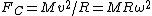# centripetal force

(redirected from Centripedal force)
Also found in: Dictionary, Thesaurus, Medical.
Related to Centripedal force: centripetal acceleration

## centripetal force

a force that acts inwards on any body that rotates or moves along a curved path and is directed towards the centre of curvature of the path or the axis of rotation

## Centripetal force

The inward force required to keep a particle or an object moving in a circular path. It can be shown that a particle moving in a circular path has an acceleration toward the center of the circle along a radius. See Acceleration

This radial acceleration, called the centripetal acceleration, is such that, if a particle has a linear or tangential velocity v when moving in a circular path of radius R, the centripetal acceleration is v2/R. If the particle undergoing the centripetal acceleration has a mass M, then by Newton's second law of motion the centripetal force FC is in the direction of the acceleration. This is expressed by the equation below,where ω is the constant angular velocity and is equal to v/R. From Newton's laws of motion it follows that the natural motion of an object is one with constant speed in a straight line, and that a force is necessary if the object is to depart from this type of motion. Whenever an object moves in a curve, a centripetal force is necessary. In circular motion the tangential speed is constant but is changing direction at the constant rate of ω, so the centripetal force along the radius is the only force involved.

## centripetal force

A force, such as gravitation, that causes a body to deviate from motion in a straight line to motion along a curved path, the force being directed toward the center of curvature of the body's motion. The force reacting against this constraint, i.e. the force equal in magnitude but opposite in direction, is the centrifugal force. The centrifugal force results from the inertia of all solid bodies, i.e. their resistance to acceleration, and unlike gravitational or electrical forces, cannot be considered a real force. The centripetal force is equal to the product of the mass of the body and its centripetal acceleration. The latter is the acceleration toward the center, and for a body moving in a circle at a constant angular velocity ω it is given by ω2r , where r is the radius.

## Centripetal Force

the force that acts on a mass point in the direction of the principal normal to the point’s trajectory and is directed toward the center of curvature. If the point moves in a circle, the centripetal force is directed toward the center of the circle. Numerically, the centripetal force that acts on a point of mass m moving with a velocity v is equal to mv2/ρ, where ρ is the radius of curvature of the point’s trajectory. Under the action of a centripetal force, the motion of a free mass point is curvilinear. During rectilinear motion, the centripetal force is equal to zero.

## centripetal force

[‚sen′trip·əd·əl ′fȯrs]
(mechanics)
The radial force required to keep a particle or object moving in a circular path, which can be shown to be directed toward the center of the circle.
Site: Follow: Share:
Open / Close